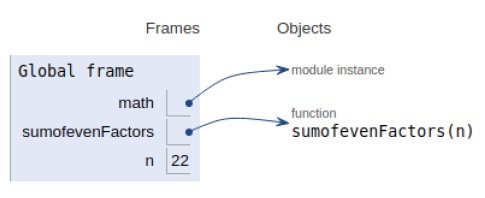# Find sum of even factors of a number in Python Program

PythonServer Side ProgrammingProgramming

Problem statement − We are given a number, we need to display the sum of all even factors of the number.

## Approach

We check whether the number is odd, then there are no even factors, so return 0.

If the number is even, we go through the computation. All other terms except 20 multiply to produce an even factor sum.

To remove all odd numbers in even factor, we ignore the 20 which is 1. After this step, we only got even factors. Note that 2 is the only even prime available to us.

Now let’s see the implementation below−

## Example

Live Demo

# math module
import math
# Returns sum of all
# factors of n.
def sumofevenFactors(n) :
# If n is odd
if (n % 2 != 0) :
return 0
# Traversal
res = 1
for i in range(2, (int)(math.sqrt(n)) + 1) :
# if i divides n
count = 0
curr_sum = 1
curr_term = 1
while (n % i == 0) :
count= count + 1
n = n // i
# here we remove the
# 2^0 that is 1. All
# other factors
if (i == 2 and count == 1) :
curr_sum = 0
curr_term = curr_term * i
curr_sum = curr_sum + curr_term
res = res * curr_sum
# when n is a prime number
if (n >= 2) :
res = res * (1 + n)
return res
# main
n = 22
print(sumofevenFactors(n))

## Output

24All the variables and functions are declared in the global scope as shown in the figure above.

## Conclusion

In this article, we have learned how we can find the sum of even factors of a number.